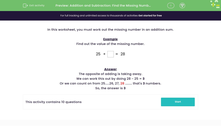# Use Addition or Subtraction to Find the Missing Number

In this worksheet, students will use addition or subtraction to work out the missing number in a problem.Key stage:  KS 1

Curriculum topic:   Number: Addition and Subtraction

Curriculum subtopic:   Understand Relationship Between Addition and Subtraction

Difficulty level:#### Worksheet Overview

Example

Find the value of the missing number.

 25 + = 28

We need to find the difference between 25 and 28.

We can turn this into a subtraction problem and work it out by doing 28 - 25 = 3

Or we can count on from 25.....26, 27, 28 ........ that's 3 numbers.

You can see that there is often more than one way of finding the answer to a maths problem. The important thing is to choose the way that works best for you.

Which of these ways do you find easier?### What is EdPlace?

We're your National Curriculum aligned online education content provider helping each child succeed in English, maths and science from year 1 to GCSE. With an EdPlace account you’ll be able to track and measure progress, helping each child achieve their best. We build confidence and attainment by personalising each child’s learning at a level that suits them.

Get started••••